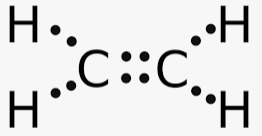c2h4 lewis structure homework Help at TutorEye

# Best Homework Help For c2h4 lewis structure

## Top Questions

erature and pressure? Record your answer in scientific notation using 3 significant figures.
View More

### What is the lewis structure for C2H4?

Each carbon atom has four valence electrons and each hydrogen atom has 1 valence electrons, and thus total 12 valence electrons in C2H4 Both the C atoms form a double bond with each other by sharing 4 electrons, and two single bonds with two H atoms and hence completing octet for both C and H atoms Each electron is represented by a dot.### Which is the correct lewis structure for ethylene C2H4?

In the Lewis structure of ethylene there are two carbon atoms and four hydrogens atoms. Each carbon atom has four valence electrons each and each hydrogen atom has 1 valence electrons, and thus total 12 valence electrons in C2H4 Both the C atoms form a double bond with each other by sharing 4 electrons, and two single bonds with two H atoms and hence completing octet for both C and H atoms. Each electron is represented by a dot.### How many total valence electrons are in the Lewis structure for C2H4?

Each carbon atom has four valence electrons and each hydrogen atom has 1 valence electrons. In the Lewis structure of ethylene there are two carbon atoms and four hydrogens atoms.
Thus valence electrons for two C atoms = 4*2=8
Valence electrons for four H atoms = 1*4=4
Total valence electrons forC2H4 = 8+4=12

### Is C2H4 tetrahedral?

In a tetrahedral geometry the carbon atom is sp3 hybridised. However, In an acetylene molecule the carbon is sp2 hybridised and that is why the molecule is planar. The molecular geometry of an acetylene molecule is trigonal planar.